# Trig Identities Flashcards

Trig Identities Flashcards
1/45 (missed) 0 0
Create Your Account To Continue Studying

As a member, you'll also get unlimited access to over 79,000 lessons in math, English, science, history, and more. Plus, get practice tests, quizzes, and personalized coaching to help you succeed.

Try it risk-free for 30 days. Cancel anytime
Complete the trig identity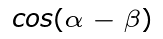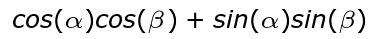Got it
Complete the trig identity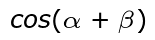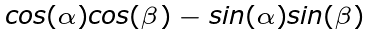Got it
Complete the trig identity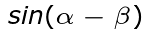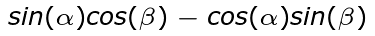Got it
Complete the trig identity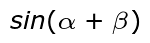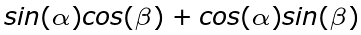Got it
How are the trig identities used?
Trig identities are used to solve problems on tests that ask you to recall specific identities, simplify a trig problem or prove trig statements.
Got it
Name this group of identities.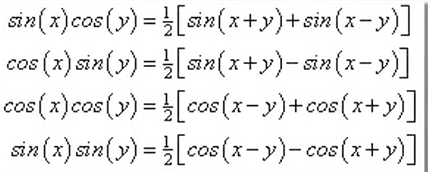Product to sum identities
Got it
What do the product to sum identities allow you to do?
Convert from the product of two trig functions to the sum or difference of two trig functions.
Got it
What is this group of trigonometric identities?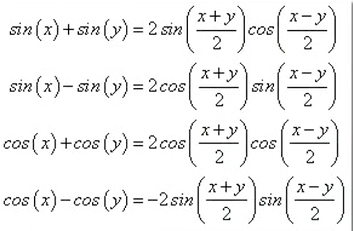Sum to product identities
Got it
What do the sum to product identities allow you to do?
Convert the sum or difference of two trig functions into the product of two trig functions.
Got it
Name this group of trigonometric identities.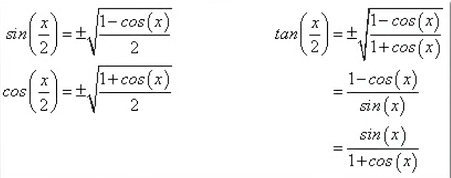Half angle identities
Got it
These identities tell you how to simplify a trig function where the angle has been halved.
Half angle identities can be used when the angle of a triangle has been cut in half.
Got it
What is this group of trigonometric identities?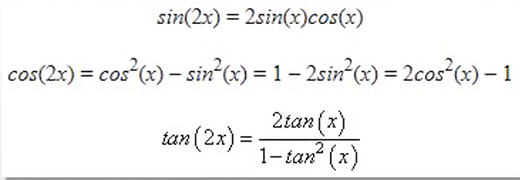Double angle identities
Got it
Which group of identities tells you how you can simplify a trig function where the angle is doubled?
The double angle identities can be used when the angle is doubled.
Got it
Name this group of trigonometric identities.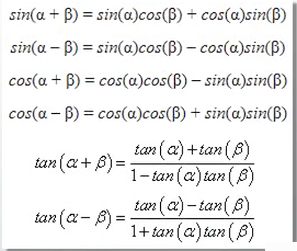The angle sum and difference identities
Got it
Which group of identities tells you how to break down a trig function that involves the addition or subtraction of two angles?
The angle sum and difference identities can be used when adding or subtracting two angles.
Got it
What is the formula for the Pythagorean theorem?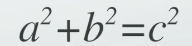Got it
What is this group of trigonometric identities?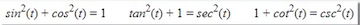The Pythagorean identities
Got it
Name this group of trigonometric identities.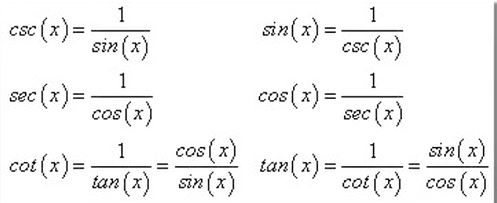Basic identities
Got it
Which group of identities include the basic definitions of the six trig functions?
Basic identities
Got it
What are trigonometric identities?
Trigonometric identities are true statements about trigonometric functions. They explain trig functions by using simpler trig terms.
Got it

or choose a specific lesson: See all lessons in this chapter
45 cards in set

## Flashcard Content Overview

This flashcard set will help you learn about trigonometric identities, which are true statements or definitions of different trigonometric functions, or how angles and sides of a triangle relate to each other. There are seven different groups of common trigonometric identities. These groups allow you to develop a better understanding of when to use a specific identity as you learn what each group can do. For example, some groups involve double angles, while others include half angles. Some identities allow you to convert from multiplication to addition or subtraction or vice versa. There are even identities that involve the Pythagorean theorem.

So, why do you need these trigonometric identities? Well, trig identities allow you to solve problems that involve simplifying a trigonometry problem. They can also help you prove various trigonometric statements. If you are scratching your head in confusion, don't worry - after you study this set of flashcards will you will have a good understanding of what trigonometric identities are and how they are used.

Front
Back
What are trigonometric identities?
Trigonometric identities are true statements about trigonometric functions. They explain trig functions by using simpler trig terms.
Which group of identities include the basic definitions of the six trig functions?
Basic identities
Name this group of trigonometric identities.Basic identities
What is this group of trigonometric identities?The Pythagorean identities
What is the formula for the Pythagorean theorem?Which group of identities tells you how to break down a trig function that involves the addition or subtraction of two angles?
The angle sum and difference identities can be used when adding or subtracting two angles.
Name this group of trigonometric identities.The angle sum and difference identities
Which group of identities tells you how you can simplify a trig function where the angle is doubled?
The double angle identities can be used when the angle is doubled.
What is this group of trigonometric identities?Double angle identities
These identities tell you how to simplify a trig function where the angle has been halved.
Half angle identities can be used when the angle of a triangle has been cut in half.
Name this group of trigonometric identities.Half angle identities
What do the sum to product identities allow you to do?
Convert the sum or difference of two trig functions into the product of two trig functions.
What is this group of trigonometric identities?Sum to product identities
What do the product to sum identities allow you to do?
Convert from the product of two trig functions to the sum or difference of two trig functions.
Name this group of identities.Product to sum identities
How are the trig identities used?
Trig identities are used to solve problems on tests that ask you to recall specific identities, simplify a trig problem or prove trig statements.
Complete the trig identityComplete the trig identityComplete the trig identityComplete the trig identityComplete the trig identity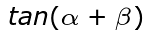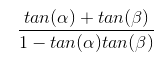Complete the trig identity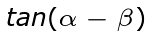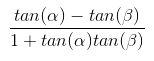Complete the trig identity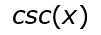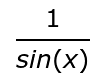Complete the trig identity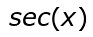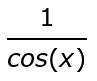Complete the trig identity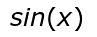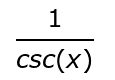Complete the trig identity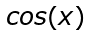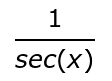Complete the trig identity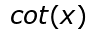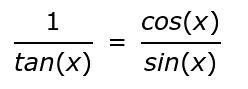Complete the trig identity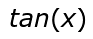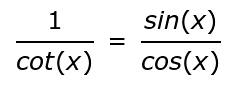Complete the trig identity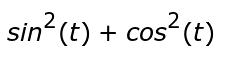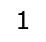Complete the trig identity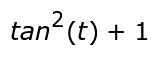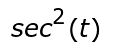Complete the trig identity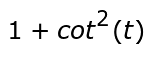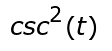Complete the trig identity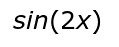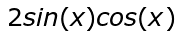Complete the trig identity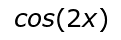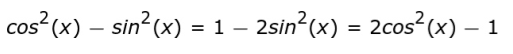Complete the trig identity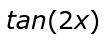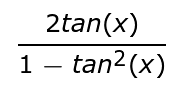Complete the trig identity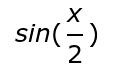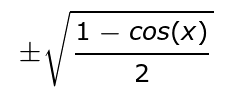Complete the trig identity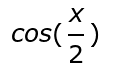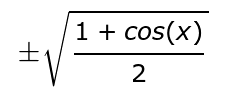Complete the trig identity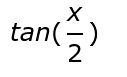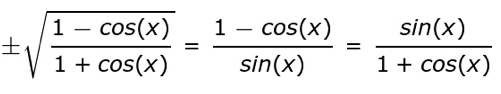Complete the trig identity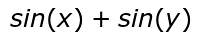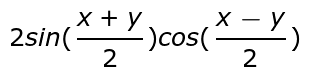Complete the trig identity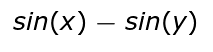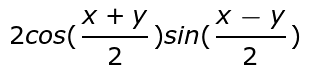Complete the trig identity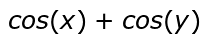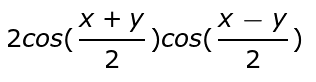Complete the trig identity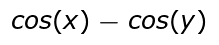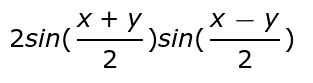Complete the trig identity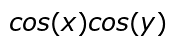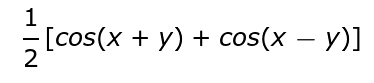Complete the trig identity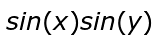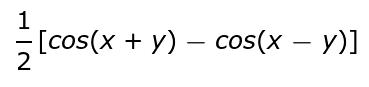Complete the trig identity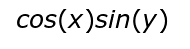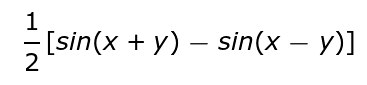Complete the trig identity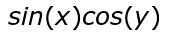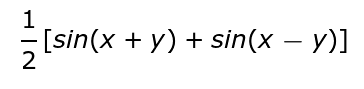To unlock this flashcard set you must be a Study.com Member.